Documentation

### This is machine translation

Mouseover text to see original. Click the button below to return to the English version of the page.

## Pattern Analysis of the Symmetric Parabolic Reflector

This example investigates the effect of feed position and reflector surface geometry on the far-field radiation pattern of a half-wavelength dipole-fed symmetric parabolic reflector. The symmetric parabolic reflector also commonly referred to as a 'dish' is a simple and widely used high gain antenna. These antennas are commonly used for satellite communications, in both civilian and military applications. The high gain of these antennas is achieved due to the electrical size of the antenna, also referred to as the aperture. The symmetric parabolic reflector has a circular aperture and its electrical size is typically reported in terms of the diameter. Depending on the application the diameter of the reflector could range from 10-30(VSAT terminals), or upwards of 100(radio astronomy).

### Reflector Parameters

For this example, we will consider a common C-band downlink frequency used by satellites such as the Intelsat-30 serving the Americas region . Also, we will target a Very Small Aperture Terminal (VSAT) application and therefore, limit the diameter of the reflector to be 1.2 m. At the upper end the electrical size of the reflector will be about 15 \lambda. Finally, the F/D ratio is chosen to be 0.3.

C_band = [3.4e9 3.7e9]; vp = physconst('lightspeed'); C_band_lambda = vp./C_band; D = 1.2; D_over_lambda_C = D./C_band_lambda; F_by_D = 0.3; 

### Design Reflector

Design the reflector at the selected frequency of 3.5 GHz and adjust the parameters as needed for the example. Re-orient the parabolic reflector to have the boresight align with x-axis.

f = 3.5e9; lambda = vp/f; p = design(reflectorParabolic,f); p.Radius = D/2; p.FocalLength = F_by_D*D; p.Tilt = 90; p.TiltAxis = [0 1 0]; figure show(p) view(45,25)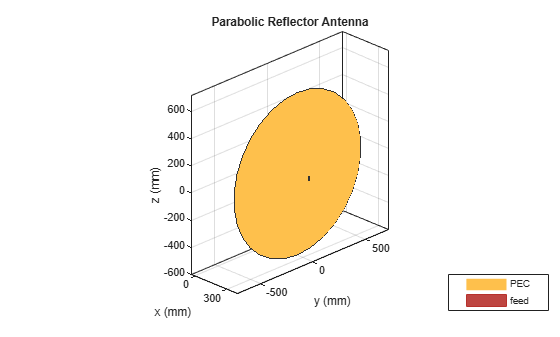### Obtain an Estimate of the Memory Requirements

Since the parabolic reflector is an electrically large structure, it is good to estimate the amount of RAM needed to solve a given structure at the frequency of design. Use the memoryEstimate function to do this.

m = memoryEstimate(p,f) 
m = '980 MB' 

### 3D Pattern

Calculate the 3D far-field directivity pattern for the forward-half plane including the boresight. In addition, we rescale the magnitude to enhance the features in the pattern using the PatternPlotOptions.

az = -90:1:90; el = -90:1:90; figure pattern(p,f,az,el)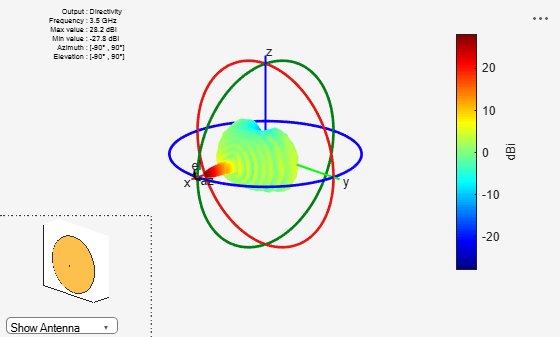Create a PatternPlotOptions object and rescale the magnitude for the plot.

patOpt = PatternPlotOptions; patOpt.MagnitudeScale = [-10 35]; figure pattern(p,f,az,el,'patternOptions',patOpt)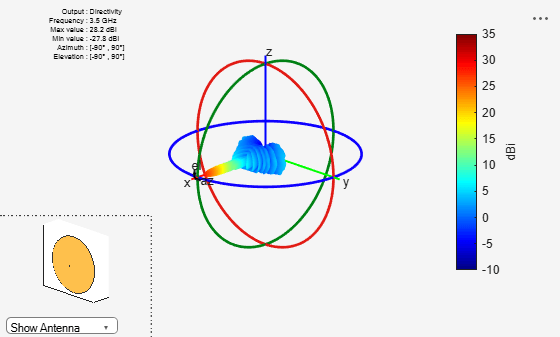### Calculating the Aperture Efficiency

The maximum gain from the parabolic reflector is achieved under uniform illumination of the aperture (amplitude, phase). A feed pattern that compensates for the spherical spreading loss with the angle off from the axis and at the same time becoming zero at the rim to avoid spillover related losses would achieve this ideal efficiency of unity . In reality we have different types of antennas that are used as feeds such as dipoles, waveguides, horns etc. Using the pattern analysis, we can numerically estimate the aperture efficiency. This calculation yields an aperture efficiency of approximately 50% for a dipole feed.

Dmax = pattern(p,f,0,0); eta_ap = (10^(Dmax/10)/(pi^2))*(lambda/D)^2 
eta_ap = 0.4971 

### Effect of Axial Displacement of the Feed

In certain applications it might be necessary to position the feed away from the focal point of the reflector. As expected such a configuration will introduce phase aberrations which will translate to a pattern degradation. Investigate the effect of an axial displacement of the feed, both - towards and away from the focus on the peak gain at boresight, i.e. (az,el) = (0,0) degrees. To do so, vary the x-coordinate of the FeedOffset property on the parabolic reflector.

feed_offset = -lambda:0.1*lambda:lambda; Dmax_offset = zeros(size(feed_offset)); for i = 1:numel(feed_offset) p.FeedOffset = [feed_offset(i),0,0]; Dmax_offset(i) = pattern(p,f,0,0); end figure plot(feed_offset./lambda,Dmax_offset,'o-','LineWidth',2) xlabel('Axial Feed Displacement (x/\lambda)') ylabel('Directivity at Boresight (dBi)') grid on title('Boresight Directivity Variation due to Axial Feed Displacement')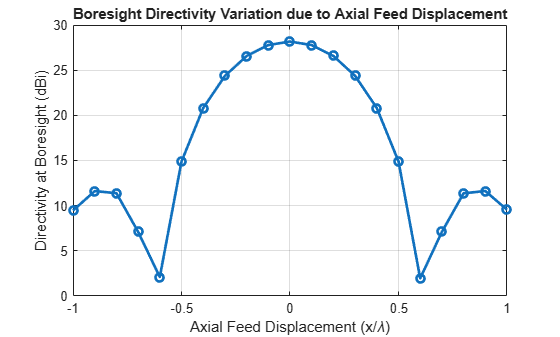### Effect of the Lateral Displacement Feed

Displacement of the feed way from the axis, laterally results in beam scan. For symmetric parabolic reflectors this effect is limited. Similar to the previous section, we continue to look at the bore sight gain variation as a function of the feed being displaced along the y-axis.

Dmax_offset = zeros(size(feed_offset)); for i = 1:numel(feed_offset) p.FeedOffset = [0,feed_offset(i),0]; Dmax_offset(i) = pattern(p,f,0,0); end figure plot(feed_offset./lambda,Dmax_offset,'o-','LineWidth',2) xlabel('Lateral Feed Displacement (y/\lambda)') ylabel('Directivity at Boresight (dBi)') grid on title('Boresight Directivity Variation due to Lateral Feed Displacement')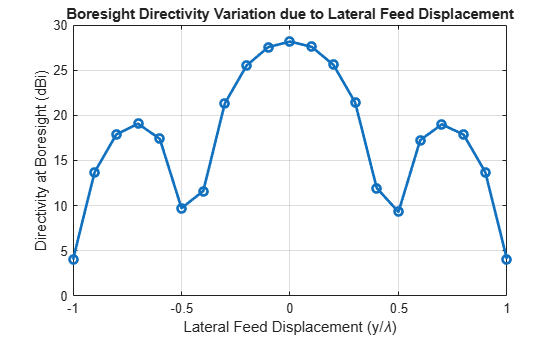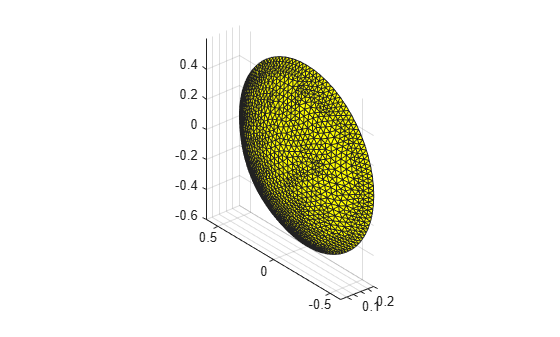### Effect of Random Surface Errors on the Reflector Surface

Ideally the surface of parabolic reflector will be perfectly smooth without any surface imperfections. Manufacturing processes and mechanical stresses result in a surface that deviates from the perfect paraboloid. Use an RMS surface error term for each co-ordinate and analytically estimate the gain degradation due to surface errors .

epsilon_rms = lambda/25; chi = (4*F_by_D)*sqrt(log(1 + 1/(4*F_by_D)^2)); Gmax_est = 10*log10(eta_ap*(pi*D/lambda)^2*exp(-1*(4*pi*chi*epsilon_rms/lambda)^2)) 
Gmax_est = 29.0023 

Next, we build a geometric model of the reflector with surface errors. To do so we isolate the mesh for the reflector alone and perturb the points on the surface with a zero-mean Gaussian random process. The standard deviation of this process is assigned to be the RMS surface error. After perturbing the points, we compute the rms surface error to confirm that the process deviation is indeed close to what we set.

p.FeedOffset = [0,0,0]; [Pt,t] = exportMesh(p); idrad = find(Pt(:,1)>=p.FocalLength); idref = find(Pt(:,1)<p.FocalLength); removeTri = []; for i = 1:size(t,1) if any(t(i,1)==idrad)||any(t(i,2)==idrad)||any(t(i,3)==idrad) removeTri = [removeTri,i]; end end tref = t; tref(removeTri,:) = []; figure em.internal.plotMesh(Pt,tref(:,1:3))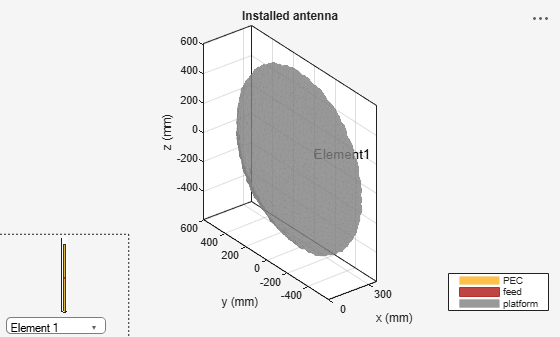Create gaussian noise for perturbing surface mesh

n = epsilon_rms*randn(numel(idref),3); Ptnoisy = Pt(idref,:) + n; rms_model_error = sqrt(mean((Pt(idref,:)-Ptnoisy).^2,1)) 
rms_model_error = 0.0034 0.0034 0.0034 

Create an STL file out of the reflector surface and make it the platform for an installed antenna analysis as shown. The excitation element is the same as before. Assign the position of the element using the feedlocation property on the parabolic reflector.

TR = triangulation(tref(:,1:3),Ptnoisy); stlwrite(TR,'noisyref.stl') pn = installedAntenna; pl = platform; exciter = p.Exciter; exciter.Tilt = 0; exciter.TiltAxis = [0 1 0]; pl.FileName = 'noisyref.stl'; pl.Units = 'm'; pn.Platform = pl; pn.Element = exciter; pn.ElementPosition = [p.FeedLocation(1),0,0]; figure show(pn)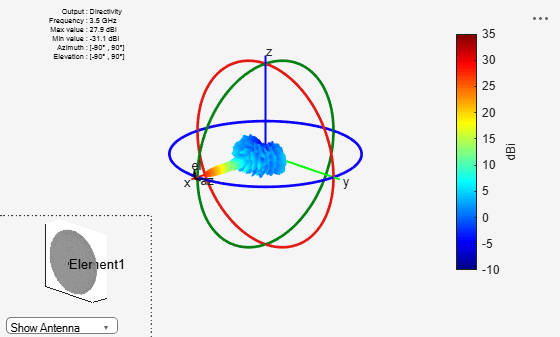### Far-field 3D Pattern for Reflector surface with Errors

The effect of the surface errors on the reflector result in a 3 dB boresight gain reduction. This effect is particularly important to consider at the Ka, Ku and higher bands

patnOpt = PatternPlotOptions; patnOpt.MagnitudeScale = [-10 35]; figure pattern(pn,f,az,el,'patternOptions',patnOpt)### References

 W. L. Stutzman, G. A. Thiele, Antenna Theory and Design, p. 307, Wiley, 3rd Edition, 2013.

 J.Ruze, "Antenna tolerance theory-a review," Proc. of IEEE, vol. 54, no.4. pp.633-640, April, 1966.